08/05, 2019

## Objetivos del práctico

• Entender los distintos tipos de errores cuadrados
• Tipo I
• Tipo II
• Tipo III
• Cuando usar cada tipo de medida de error cuadrado
• Tipo I (Diseños anidados)
• Tipo II (Diseños factoriales sin interacción)
• Tipo III (Diseños factoriales con interacción)
• Como implementarlo en R
• Solo importa en ANOVAS de dos o mas factores

## Antes de empezar

• Función Anova: Nos permite medir diferencias entre dos modelos lineales o ANOVAS
anova(aov(mpg ~ am, data =mtcars), aov(mpg ~ am + cyl, data =mtcars))
## Analysis of Variance Table
##
## Model 1: mpg ~ am
## Model 2: mpg ~ am + cyl
##   Res.Df    RSS Df Sum of Sq      F    Pr(>F)
## 1     30 720.90
## 2     29 271.36  1    449.53 48.041 1.285e-07 ***
## ---
## Signif. codes:  0 '***' 0.001 '**' 0.01 '*' 0.05 '.' 0.1 ' ' 1

Usaremos esta función para explicar los tipos de errores cuadrados

## Error tipo I (Error por defecto de R)

• Cuando usarlo: Modelos anidados, anovas de una vía
• Cuando no usarlo: Modelos factoriales (El orden de los factores importa)
aov(mpg ~ am*cyl, data = mtcars)
aov(mpg ~ cyl*am, data = mtcars)
am primero
cyl primero
term df sumsq p.value term1 df1 sumsq1 p.value1
am 1 405.15059 0.0000002 cyl 1 817.71295 0.0000000
cyl 1 449.53448 0.0000001 am 1 36.97211 0.0479300
am:cyl 1 29.43939 0.0755069 cyl:am 1 29.43939 0.0755069
Residuals 28 241.92273 NA Residuals 28 241.92273 NA

## ¿Por que cambian los valores?

Errores cuadrados secuenciales y ~ A + B + *A:B esto se desglosa así:

• Primero como explica A los datos (y ~ A)
• Luego como explica B los datos después de que A explico los datos (y ~ B|A)
• Finalmente como explica la interacción dado lo que ya explican ambas variables en conjunto (y ~ A:B| A + B)
• Por eso es bueno para sistemas anidados

broom::tidy(aov(uptake ~ Treatment*Type, data = CO2))
term df sumsq meansq statistic p.value
Treatment 1 988.1144 988.11440 15.41641 0.0001817
Type 1 3365.5344 3365.53440 52.50856 0.0000000
Treatment:Type 1 225.7296 225.72964 3.52180 0.0642128
Residuals 80 5127.5971 64.09496 NA NA

## Cuanto explica Treatment

Treatment

anova(aov(uptake ~ 1, data = CO2), aov(uptake ~ Treatment, data = CO2))
## Analysis of Variance Table
##
## Model 1: uptake ~ 1
## Model 2: uptake ~ Treatment
##   Res.Df    RSS Df Sum of Sq      F   Pr(>F)
## 1     83 9707.0
## 2     82 8718.9  1    988.11 9.2931 0.003096 **
## ---
## Signif. codes:  0 '***' 0.001 '**' 0.01 '*' 0.05 '.' 0.1 ' ' 1

## Cuanto explica Treatment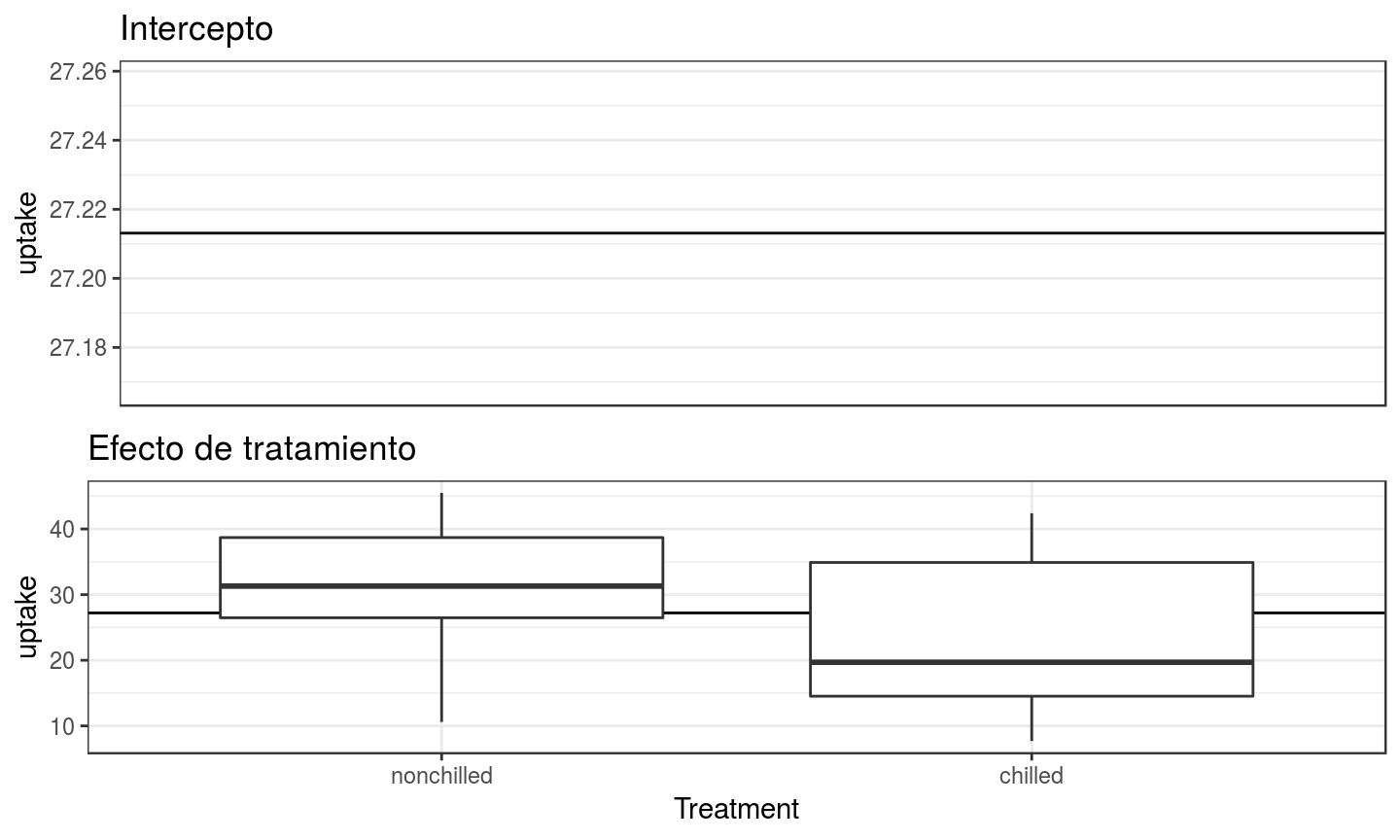## Cuanto explica Type dado Treatment

anova(aov(uptake ~ Treatment, data = CO2), aov(uptake ~ Treatment + Type, data = CO2))
## Analysis of Variance Table
##
## Model 1: uptake ~ Treatment
## Model 2: uptake ~ Treatment + Type
##   Res.Df    RSS Df Sum of Sq      F    Pr(>F)
## 1     82 8718.9
## 2     81 5353.3  1    3365.5 50.923 3.679e-10 ***
## ---
## Signif. codes:  0 '***' 0.001 '**' 0.01 '*' 0.05 '.' 0.1 ' ' 1

## Cuanto explica Type dado Treatment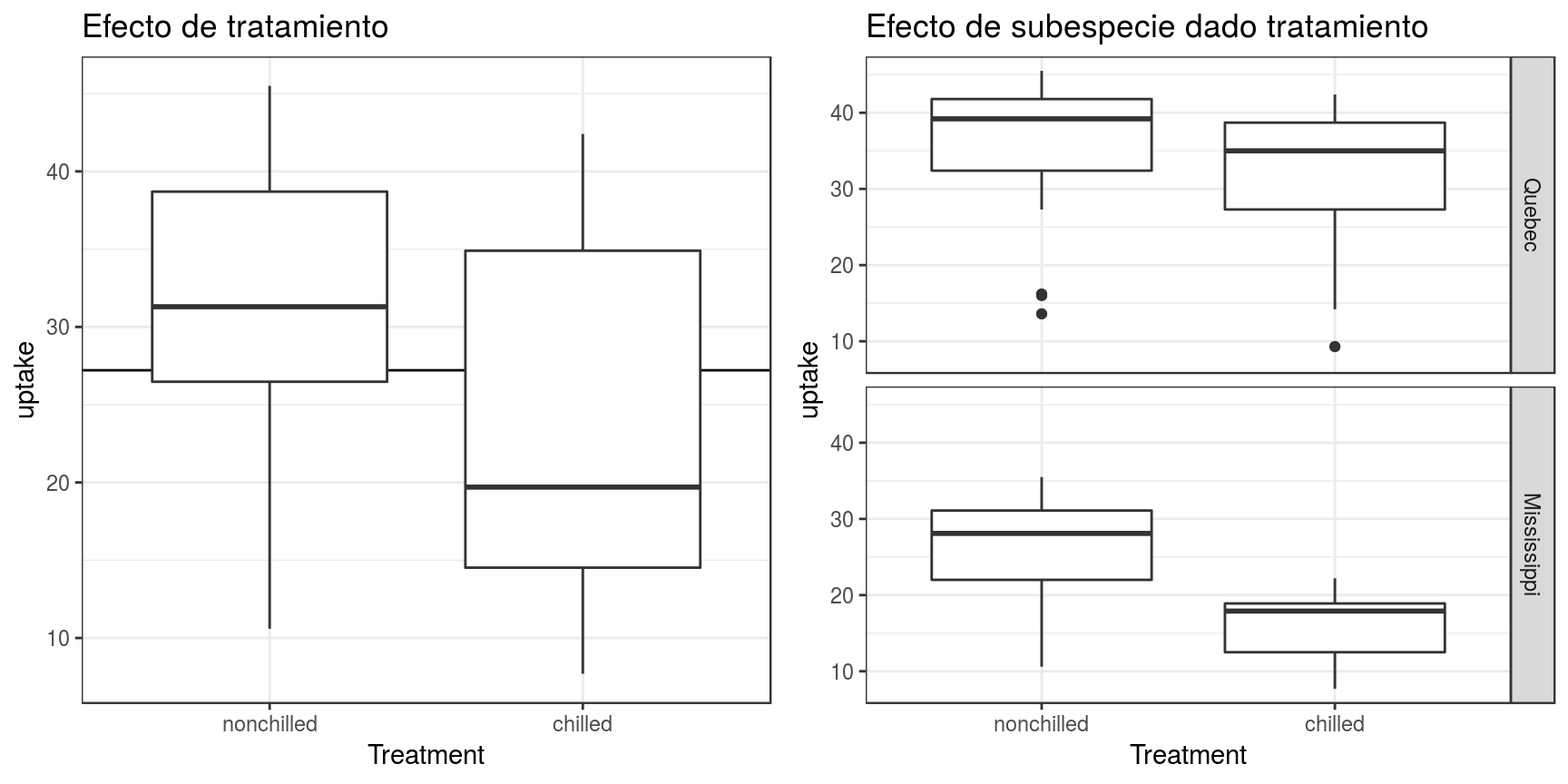## Cuanto explica la interaccion dadas ambas variables

anova(aov(uptake ~ Treatment + Type, data = CO2), aov(uptake ~
Treatment + Type + Treatment:Type, data = CO2))
## Analysis of Variance Table
##
## Model 1: uptake ~ Treatment + Type
## Model 2: uptake ~ Treatment + Type + Treatment:Type
##   Res.Df    RSS Df Sum of Sq      F  Pr(>F)
## 1     81 5353.3
## 2     80 5127.6  1    225.73 3.5218 0.06421 .
## ---
## Signif. codes:  0 '***' 0.001 '**' 0.01 '*' 0.05 '.' 0.1 ' ' 1

## Cuanto explica la interaccion dadas ambas variables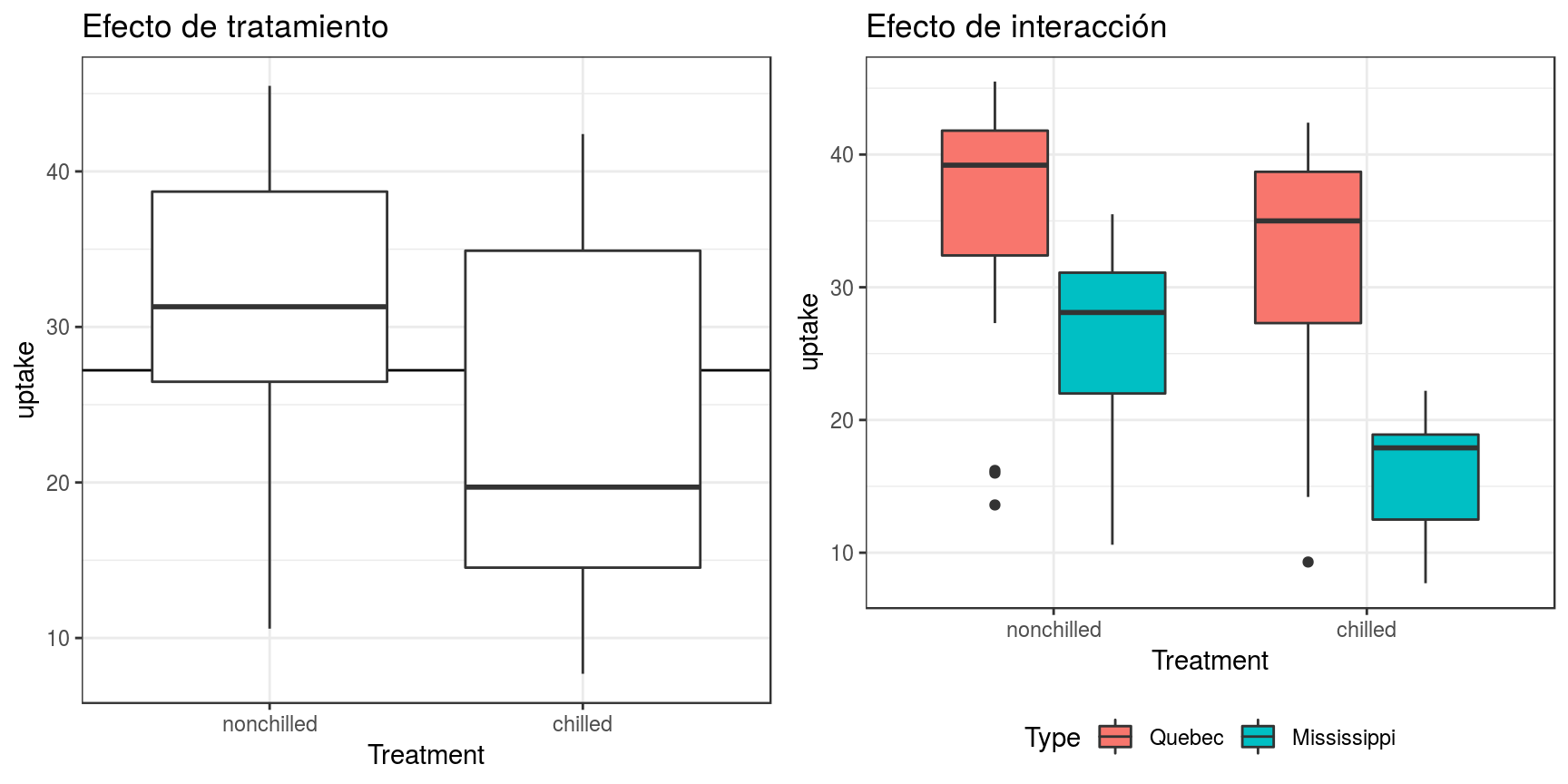## Error tipo II

• Cuando usarlo: Cuando no hay interacciones (el más poderoso), anovas de una vía (orden no importa)
• Cuando no usarlo: Modelos factoriales interacciones fuertes
library(car)
Anova(aov(mpg ~ am*cyl, data = mtcars), type = 2)
## Anova Table (Type II tests)
##
## Response: mpg
##           Sum Sq Df F value    Pr(>F)
## am         36.97  1  4.2791   0.04793 *
## cyl       449.53  1 52.0289 7.503e-08 ***
## am:cyl     29.44  1  3.4073   0.07551 .
## Residuals 241.92 28
## ---
## Signif. codes:  0 '***' 0.001 '**' 0.01 '*' 0.05 '.' 0.1 ' ' 1
Anova(aov(mpg ~ cyl*am, data = mtcars), type = 2)
## Anova Table (Type II tests)
##
## Response: mpg
##           Sum Sq Df F value    Pr(>F)
## cyl       449.53  1 52.0289 7.503e-08 ***
## am         36.97  1  4.2791   0.04793 *
## cyl:am     29.44  1  3.4073   0.07551 .
## Residuals 241.92 28
## ---
## Signif. codes:  0 '***' 0.001 '**' 0.01 '*' 0.05 '.' 0.1 ' ' 1

## ¿Cual es la diferencia con el tipo 1?

tipo 2
tipo 1
term sumsq df p.value df1 sumsq1 p.value1
am 36.97211 1 0.0479300 1 817.71295 0.0000000
cyl 449.53448 1 0.0000001 1 36.97211 0.0479300
am:cyl 29.43939 1 0.0755069 1 29.43939 0.0755069
Residuals 241.92273 28 NA 28 241.92273 NA

## Orden de los errores

Errores cuadrados de y ~ A + B + *A:B se desglosan así:

• Primero como explica A los datos despues de que B explico los datos (y ~ A|B)
• Luego como explica B los datos después de que A explico los datos (y ~ B|A)
• Finalmente como explica la interacción dado lo que ya explican ambas variables en conjunto (y ~ A:B| A + B)
• Por le da última prioridad a las interacciones
• Bueno si nos interesan efectos principales
• Malo si nos interesan más las interacciones

Anova(aov(mpg ~ am*cyl, data = mtcars), type = 2)
## Anova Table (Type II tests)
##
## Response: mpg
##           Sum Sq Df F value    Pr(>F)
## am         36.97  1  4.2791   0.04793 *
## cyl       449.53  1 52.0289 7.503e-08 ***
## am:cyl     29.44  1  3.4073   0.07551 .
## Residuals 241.92 28
## ---
## Signif. codes:  0 '***' 0.001 '**' 0.01 '*' 0.05 '.' 0.1 ' ' 1

## Cuanto explica am, cuando ya tomamos en cuenta cyl

anova(aov(mpg ~ cyl, data = mtcars), aov(mpg ~ am + cyl, data = mtcars))
## Analysis of Variance Table
##
## Model 1: mpg ~ cyl
## Model 2: mpg ~ am + cyl
##   Res.Df    RSS Df Sum of Sq      F  Pr(>F)
## 1     30 308.33
## 2     29 271.36  1    36.972 3.9511 0.05635 .
## ---
## Signif. codes:  0 '***' 0.001 '**' 0.01 '*' 0.05 '.' 0.1 ' ' 1

## Cuanto explica am, cuando ya tomamos en cuenta cyl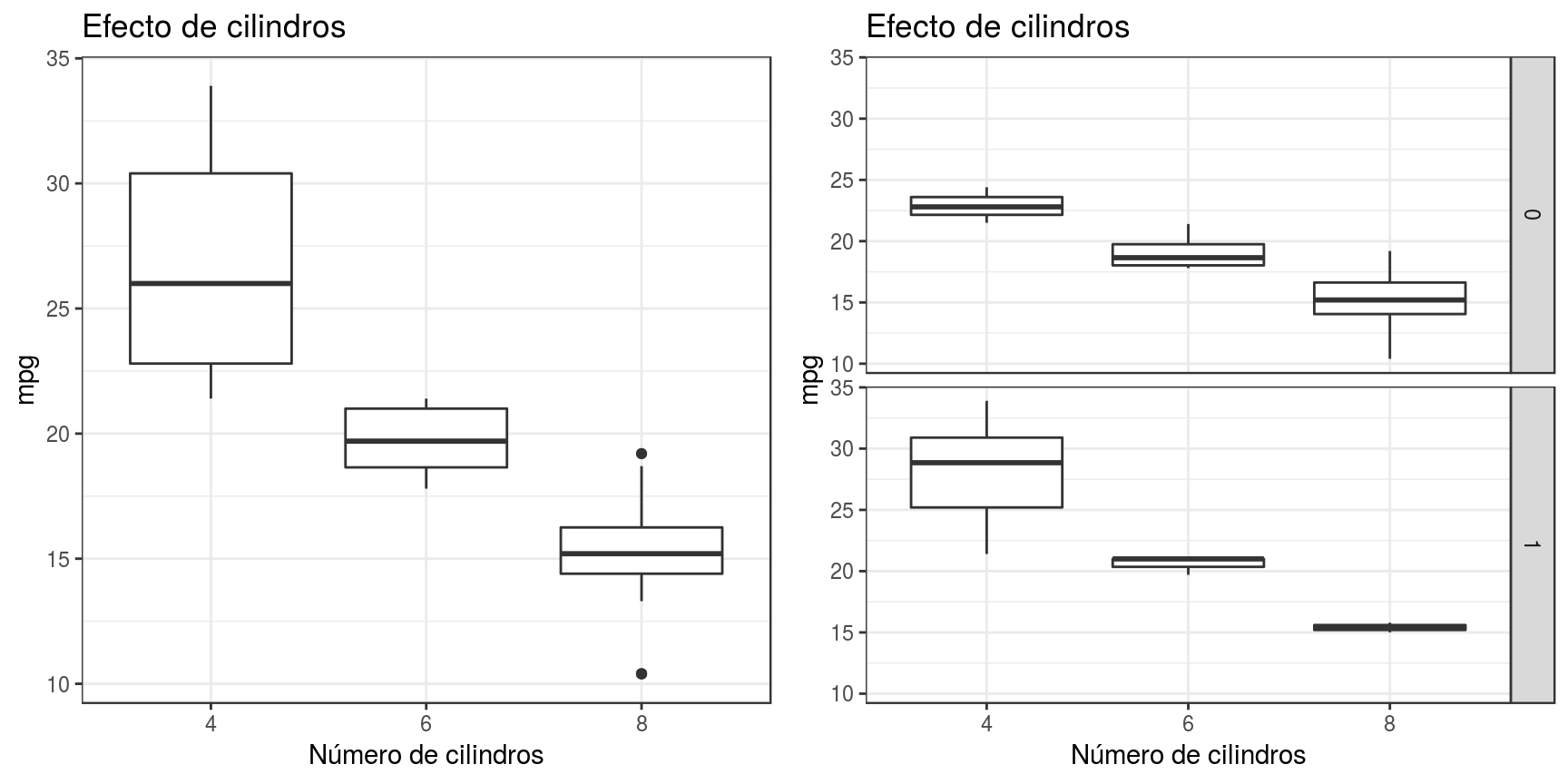## Cuanto explica cyl, cuando ya tomamos en cuenta am

anova(aov(mpg ~ am, data = mtcars), aov(mpg ~ am + cyl, data = mtcars))
## Analysis of Variance Table
##
## Model 1: mpg ~ am
## Model 2: mpg ~ am + cyl
##   Res.Df    RSS Df Sum of Sq      F    Pr(>F)
## 1     30 720.90
## 2     29 271.36  1    449.53 48.041 1.285e-07 ***
## ---
## Signif. codes:  0 '***' 0.001 '**' 0.01 '*' 0.05 '.' 0.1 ' ' 1

## Cuanto explica cyl, cuando ya tomamos en cuenta am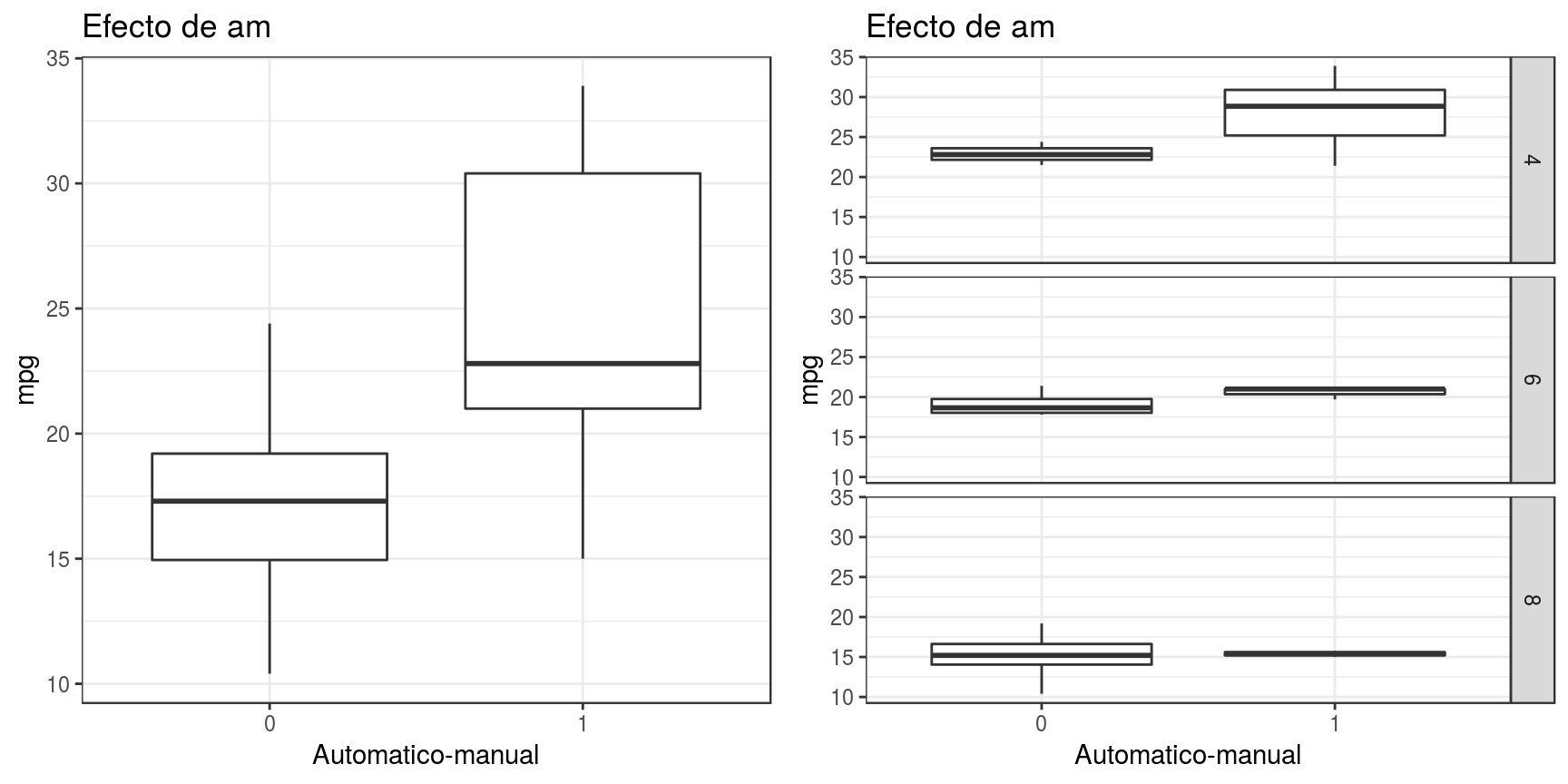## Cuanto explica interacción, cuando ya tomamos en cuenta am y cyl

anova(aov(mpg ~ am + cyl, data = mtcars), aov(mpg ~ am *cyl, data = mtcars))
## Analysis of Variance Table
##
## Model 1: mpg ~ am + cyl
## Model 2: mpg ~ am * cyl
##   Res.Df    RSS Df Sum of Sq      F  Pr(>F)
## 1     29 271.36
## 2     28 241.92  1    29.439 3.4073 0.07551 .
## ---
## Signif. codes:  0 '***' 0.001 '**' 0.01 '*' 0.05 '.' 0.1 ' ' 1

## Cuanto explica interacción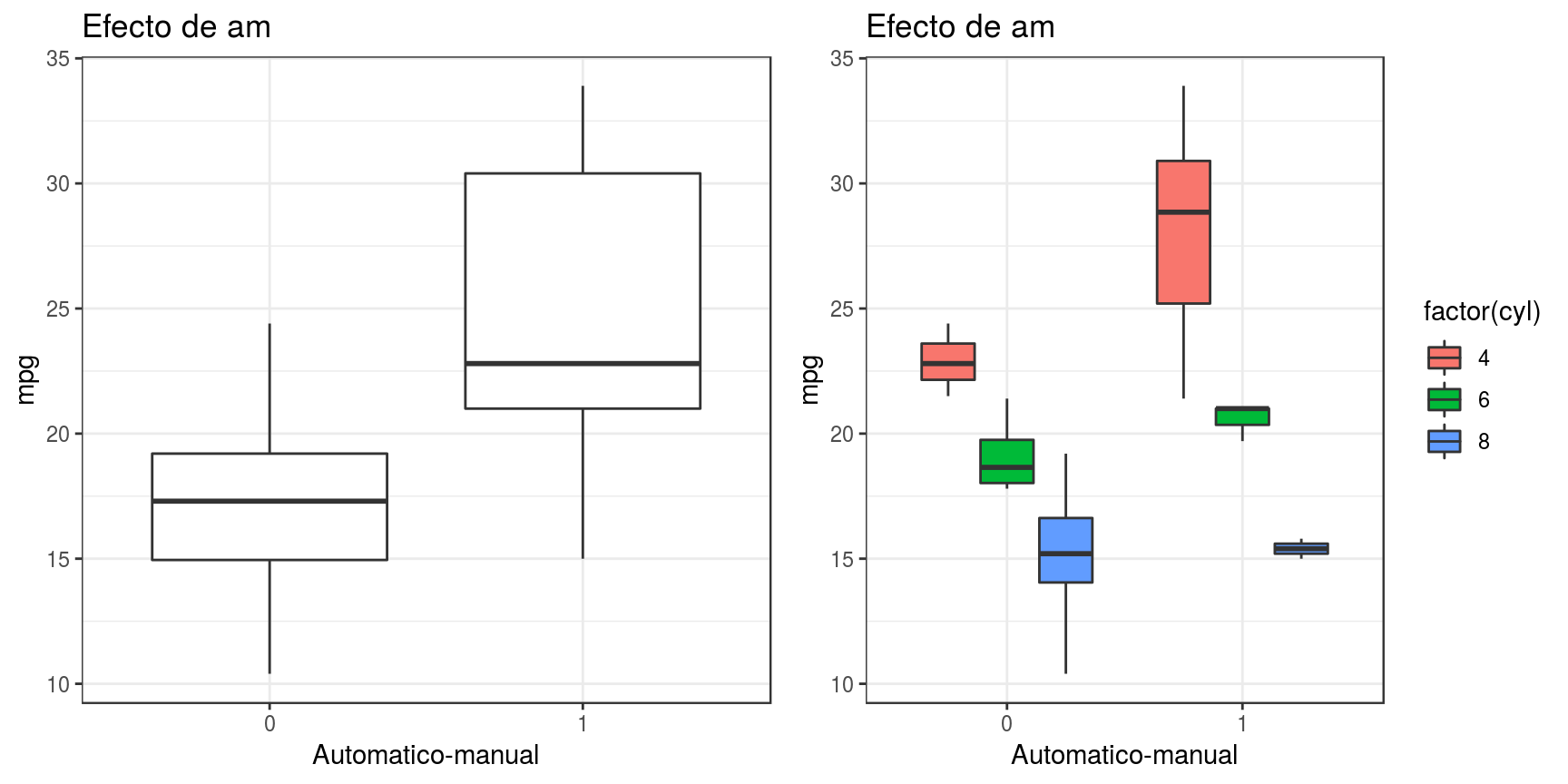## Error tipo III

• Cuando usarlo: Cuando hay interacciones, diseños desbalanceados, anovas de una vía (orden no importa)
• Cuando no usarlo: Cuando las interacciones son fuertes no permite detectar efectos principales
Anova(aov(mpg ~ am*cyl, data = mtcars), type = 3)
## Anova Table (Type III tests)
##
## Response: mpg
##             Sum Sq Df F value    Pr(>F)
## (Intercept) 810.20  1 93.7719 1.948e-10 ***
## am           48.28  1  5.5879 0.0252577 *
## cyl         167.65  1 19.4033 0.0001407 ***
## am:cyl       29.44  1  3.4073 0.0755069 .
## Residuals   241.92 28
## ---
## Signif. codes:  0 '***' 0.001 '**' 0.01 '*' 0.05 '.' 0.1 ' ' 1

## ¿Cual es la diferencia con el tipo 2?

## Anova Table (Type II tests)
##
## Response: mpg
##           Sum Sq Df F value    Pr(>F)
## am         36.97  1  4.2791   0.04793 *
## cyl       449.53  1 52.0289 7.503e-08 ***
## am:cyl     29.44  1  3.4073   0.07551 .
## Residuals 241.92 28
## ---
## Signif. codes:  0 '***' 0.001 '**' 0.01 '*' 0.05 '.' 0.1 ' ' 1
## Anova Table (Type III tests)
##
## Response: mpg
##             Sum Sq Df F value    Pr(>F)
## (Intercept) 810.20  1 93.7719 1.948e-10 ***
## am           48.28  1  5.5879 0.0252577 *
## cyl         167.65  1 19.4033 0.0001407 ***
## am:cyl       29.44  1  3.4073 0.0755069 .
## Residuals   241.92 28
## ---
## Signif. codes:  0 '***' 0.001 '**' 0.01 '*' 0.05 '.' 0.1 ' ' 1

## Orden de los errores

Errores cuadrados dey ~ A + B + *A:B se desglosan así:

• Primero como explica A los datos despues de que B y las interacciones lo explicaron (y ~ A|B + A:B)
• Luego como explica B los datos después de que A e interacciones explicaran los datos (y ~ B|A + A:B)
• Finalmente como explica la interacción dado lo que ya explican ambas variables en conjunto (y ~ A:B| A + B)
• Solo le da a cada variable lo que explica por si sola
• Malo si nos interesan mas los efectos principales
• Bueno si nos interesan más las interacciones

Anova(aov(mpg ~ am*cyl, data = mtcars), type = 3)
## Anova Table (Type III tests)
##
## Response: mpg
##             Sum Sq Df F value    Pr(>F)
## (Intercept) 810.20  1 93.7719 1.948e-10 ***
## am           48.28  1  5.5879 0.0252577 *
## cyl         167.65  1 19.4033 0.0001407 ***
## am:cyl       29.44  1  3.4073 0.0755069 .
## Residuals   241.92 28
## ---
## Signif. codes:  0 '***' 0.001 '**' 0.01 '*' 0.05 '.' 0.1 ' ' 1

## Cuanto explica am, cuando ya tomamos en cuenta cyl y las interacciones

anova(aov(mpg ~ cyl, data = mtcars), aov(mpg ~ am + am:cyl, data = mtcars))
## Analysis of Variance Table
##
## Model 1: mpg ~ cyl
## Model 2: mpg ~ am + am:cyl
##   Res.Df    RSS Df Sum of Sq F Pr(>F)
## 1     30 308.33
## 2     29 409.57  1   -101.23

## Cuanto explica cyl, cuando ya tomamos en cuenta am y las interacciones

anova(aov(mpg ~ am, data = mtcars), aov(mpg ~ cyl + am:cyl, data = mtcars))
## Analysis of Variance Table
##
## Model 1: mpg ~ am
## Model 2: mpg ~ cyl + am:cyl
##   Res.Df   RSS Df Sum of Sq      F    Pr(>F)
## 1     30 720.9
## 2     29 290.2  1    430.69 43.039 3.466e-07 ***
## ---
## Signif. codes:  0 '***' 0.001 '**' 0.01 '*' 0.05 '.' 0.1 ' ' 1

## Cuanto explica interacción, cuando ya tomamos en cuenta am y cyl

anova(aov(mpg ~ am + cyl, data = mtcars), aov(mpg ~ am *cyl, data = mtcars))
## Analysis of Variance Table
##
## Model 1: mpg ~ am + cyl
## Model 2: mpg ~ am * cyl
##   Res.Df    RSS Df Sum of Sq      F  Pr(>F)
## 1     29 271.36
## 2     28 241.92  1    29.439 3.4073 0.07551 .
## ---
## Signif. codes:  0 '***' 0.001 '**' 0.01 '*' 0.05 '.' 0.1 ' ' 1

## Exploren ANOVAS

Crearemos base de datos simulada con la funcion SimulData:

SimulData <- function(MeanA1B1 = 20, MeanA1B2 = 20, MeanA1B3 = 20,
MeanA2B1 = 40, MeanA2B2 = 40, MeanA2B3 = 40, N = 5) {
data.frame(A = rep(c("A1", "A2"), each = 3 * N), B = rep(rep(c("B1",
"B2", "B3"), each = N), times = 2), y = (c(rnorm(mean = MeanA1B1,
n = N, sd = 5), rnorm(mean = MeanA1B2, n = N, sd = 5),
rnorm(mean = MeanA1B3, n = N, sd = 5), rnorm(mean = MeanA2B1,
n = N, sd = 5), rnorm(mean = MeanA2B2, n = N, sd = 5),
rnorm(mean = MeanA2B3, n = N, sd = 5))))
}

## Exploren ANOVAS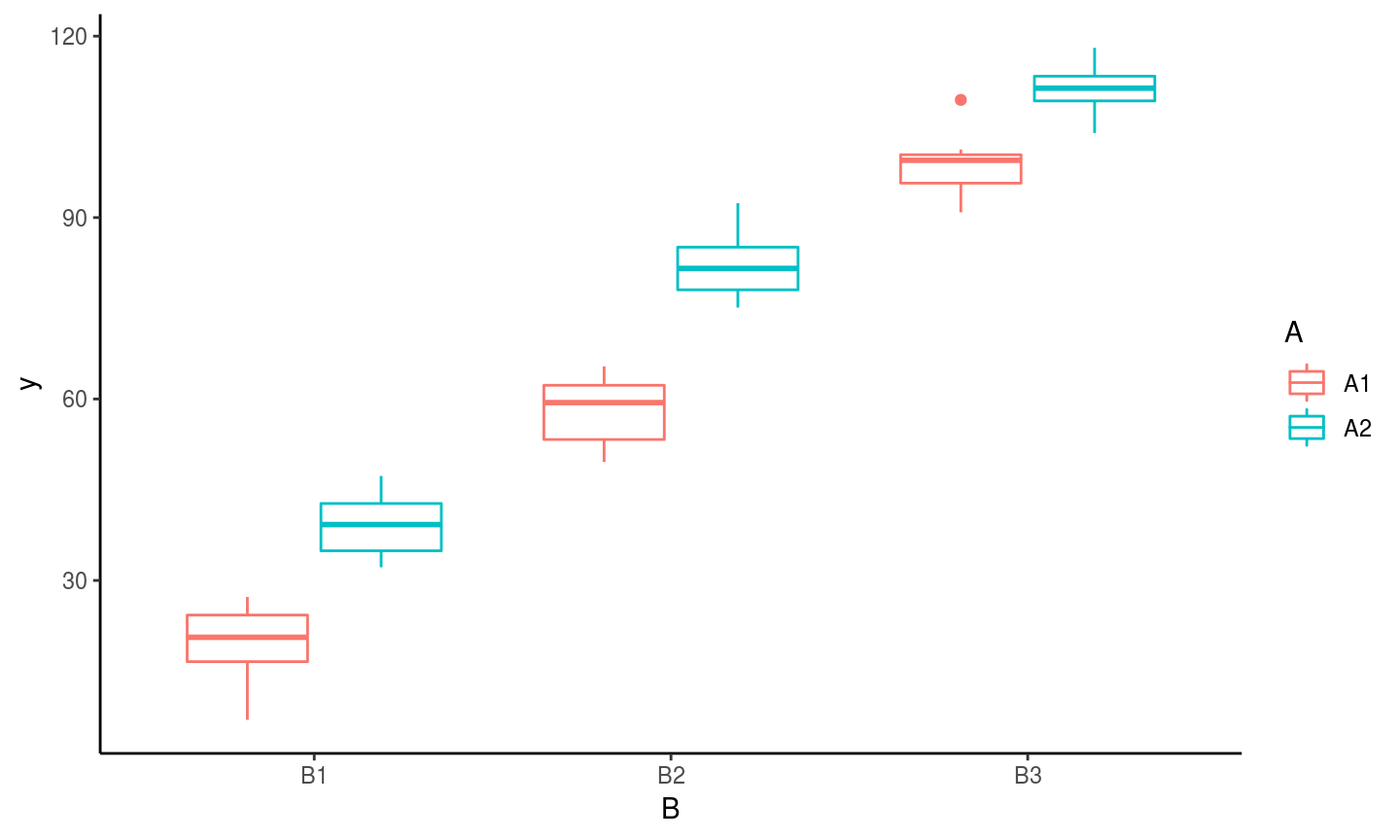## Exploren ANOVAS

Graficaremos con la funcion InterPlot

InterPlot <- function(DF) {
require(tidyverse)
DF <- group_by(DF, A, B) %>% summarize(MeanY = mean(y), SDy = sd(y))
ggplot(DF, aes(x = B, y = MeanY, group = A)) + geom_point(aes(color = A)) +
geom_errorbar(aes(color = A, ymax = MeanY + SDy, ymin = MeanY -
SDy)) + geom_line(aes(color = A)) + theme_classic()
}

## Exploren ANOVAS

InterPlot(DF)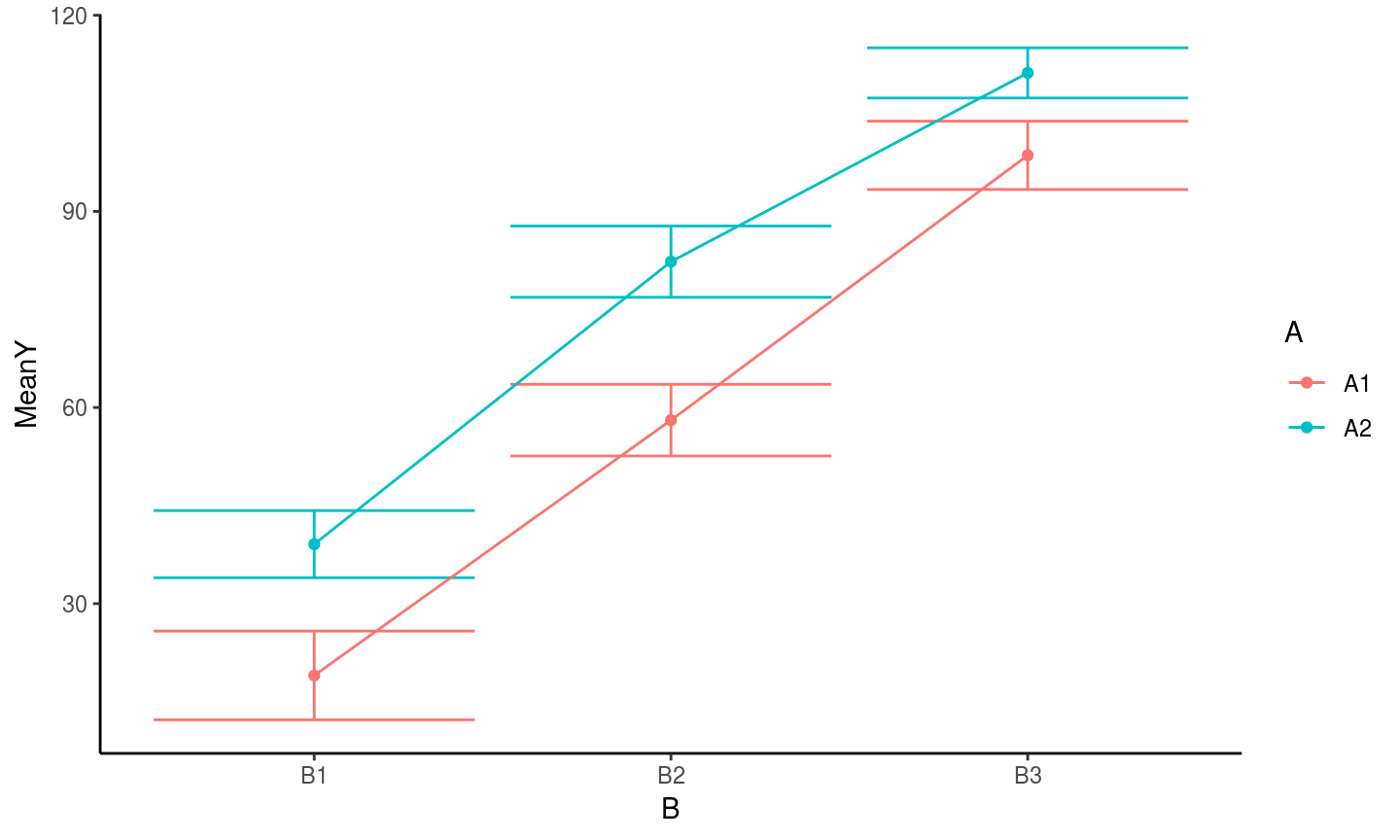## Exploren ANOVAS

anova(aov(y ~ A*B, data = DF))
## Analysis of Variance Table
##
## Response: y
##           Df Sum Sq Mean Sq  F value    Pr(>F)
## A          1   5403  5402.9 186.0663 < 2.2e-16 ***
## B          2  57634 28816.9 992.4053 < 2.2e-16 ***
## A:B        2    348   173.9   5.9887  0.004477 **
## Residuals 54   1568    29.0
## ---
## Signif. codes:  0 '***' 0.001 '**' 0.01 '*' 0.05 '.' 0.1 ' ' 1
anova(aov(y ~ B*A, data = DF))
## Analysis of Variance Table
##
## Response: y
##           Df Sum Sq Mean Sq  F value    Pr(>F)
## B          2  57634 28816.9 992.4053 < 2.2e-16 ***
## A          1   5403  5402.9 186.0663 < 2.2e-16 ***
## B:A        2    348   173.9   5.9887  0.004477 **
## Residuals 54   1568    29.0
## ---
## Signif. codes:  0 '***' 0.001 '**' 0.01 '*' 0.05 '.' 0.1 ' ' 1
Anova(aov(y ~ A*B, data = DF), type = 2)
## Anova Table (Type II tests)
##
## Response: y
##           Sum Sq Df  F value    Pr(>F)
## A           5403  1 186.0663 < 2.2e-16 ***
## B          57634  2 992.4053 < 2.2e-16 ***
## A:B          348  2   5.9887  0.004477 **
## Residuals   1568 54
## ---
## Signif. codes:  0 '***' 0.001 '**' 0.01 '*' 0.05 '.' 0.1 ' ' 1
Anova(aov(y ~ A*B, data = DF), type = 3)
## Anova Table (Type III tests)
##
## Response: y
##             Sum Sq Df  F value    Pr(>F)
## (Intercept)   3615  1 124.5079 1.226e-15 ***
## A             2016  1  69.4315 2.877e-11 ***
## B            31654  2 545.0532 < 2.2e-16 ***
## A:B            348  2   5.9887  0.004477 **
## Residuals     1568 54
## ---
## Signif. codes:  0 '***' 0.001 '**' 0.01 '*' 0.05 '.' 0.1 ' ' 1